Courses

# Test: Fixed Bias & Emitter Bias

## 10 Questions MCQ Test Analog Circuits | Test: Fixed Bias & Emitter Bias

Description
This mock test of Test: Fixed Bias & Emitter Bias for Electronics and Communication Engineering (ECE) helps you for every Electronics and Communication Engineering (ECE) entrance exam. This contains 10 Multiple Choice Questions for Electronics and Communication Engineering (ECE) Test: Fixed Bias & Emitter Bias (mcq) to study with solutions a complete question bank. The solved questions answers in this Test: Fixed Bias & Emitter Bias quiz give you a good mix of easy questions and tough questions. Electronics and Communication Engineering (ECE) students definitely take this Test: Fixed Bias & Emitter Bias exercise for a better result in the exam. You can find other Test: Fixed Bias & Emitter Bias extra questions, long questions & short questions for Electronics and Communication Engineering (ECE) on EduRev as well by searching above.
QUESTION: 1

### Whichof the following is the correct relatioship between IB and IE?

Solution:

For a BJT, the collector and emitter current are related as IE = (β + 1) IB.

QUESTION: 2

### For best operation of a BJT, which region must the operating point be set at?

Solution:

Operating point for a BJT must always be set in the active region to ensure proper functioning. Setting up of Q-point in any other region may lead to reduced functionality.

QUESTION: 3

### From the given circuit, what is the value of IBQ?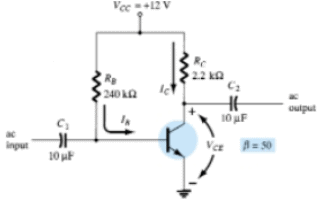Solution:

We know that IBQ = (VCC – VBE)/RB = 11.3/240 uA = 47.08 uA.

QUESTION: 4

From the given circuit, what is the value of VCEQ?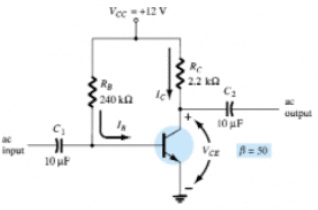Solution:

VCEQ = VCC – ICRC. Hence, VCEQ = 6.83 V.

QUESTION: 5

From the given circuit, what is the value of VBC?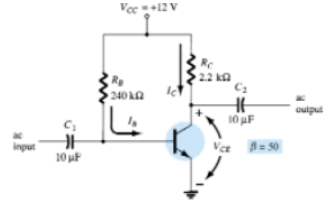Solution:

VBC = VB – VC = VBE – VCE = -6.13 V.

QUESTION: 6

From the given circuit, what is the value of the saturation collector current?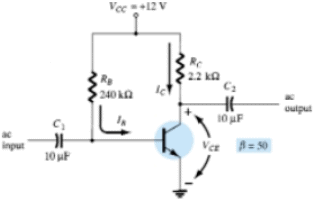Solution:

Saturation collector current = VCC/RC = 5.45 mA.

QUESTION: 7

In the given circuit, what is the value of IC?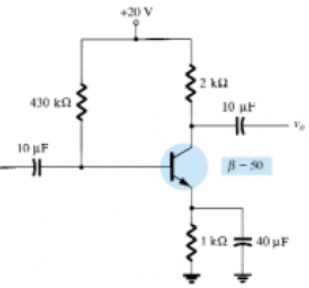Solution:

β IB = 2.01 mA.

QUESTION: 8

In the given circuit, what is the value of VCE?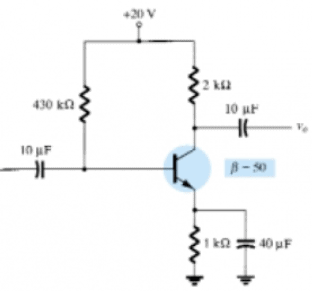Solution:

VCE = VCC – ICRC = 13.97 V.

QUESTION: 9

In the given circuit, what is the value of VE?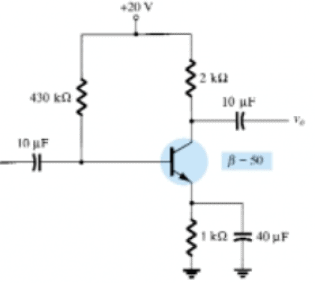Solution:

VE = VC – VCE = 2.01 V.

QUESTION: 10

In the given circuit, what is the value of saturation collector current?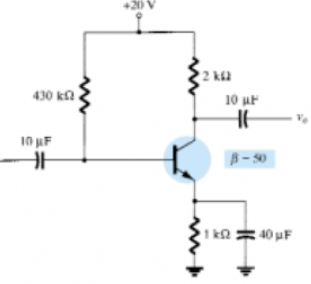Solution:

Saturation collector current = VCC/(RC + RE) = 6.67 mA.